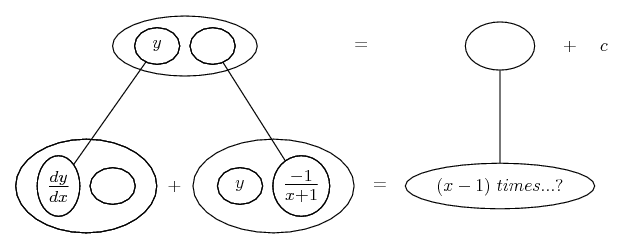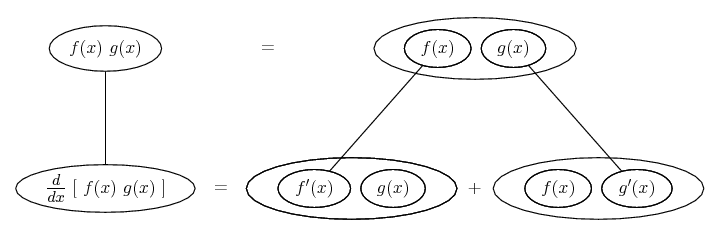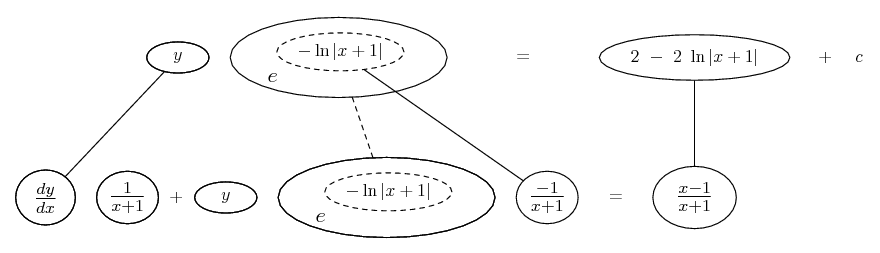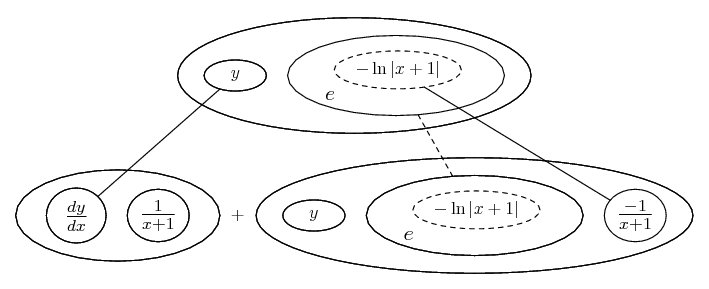# Thread: Solving differential equation

1. ## Solving differential equation

The equation I have is:

(x+1)dy/dx - y = x^2 - 1

I basically need to rearrange it to find the integrating factor, then I need to solve it to find the general solution. Also how do I solve the particular solution? I need to find it when y(0) = 0.

Thanks for any help

LooN

2. The equation i read as being

$\displaystyle (x + 1)\frac{{dy}}{{dx}} - y = x^2 - 1$

Linear differential equation needs to be in the form $\displaystyle y' + P(x)y = Q(x)$

So divide through by x+1 to get dy/dx on its own.

$\displaystyle \begin{array}{l} \frac{{dy}}{{dx}} - \left( {\frac{1}{{x + 1}}} \right)y = \frac{{x^2 - 1}}{{x + 1}} \\ \frac{{dy}}{{dx}} - \left( {\frac{1}{{x + 1}}} \right)y = \frac{{(x - 1)(x + 1)}}{{x + 1}} \\ \frac{{dy}}{{dx}} - \left( {\frac{1}{{x + 1}}} \right)y = x - 1 \\ \end{array}$

The integrating factor is in the form $\displaystyle I(x) = e^{\int {P(x)dx} }$

$\displaystyle \begin{array}{l} P(x) = \frac{1}{{x + 1}} \\ Q(x) = x - 1 \\ \end{array}$

So integrating factor will be
$\displaystyle I(x) = e^{\int {P(x)dx} } = e^{\int {\frac{1}{{x + 1}}dx} } = e^{\ln (x + 1)}$

From this you need to multiply the differential equation in linear form by I(x)
ie $\displaystyle I(x)y' + I(x)P(x)y = I(x)Q(x)$

From here I get a little lost myself, I'm learning these also, so I'll leave it here so that maybe someone can clarify the rest.

3. ## further help

can anyone help out further with this question? Its so confusing. !bump

4. !bump

5. It is a linear differential equation that has 'standard form' ...

$\displaystyle y'= a(x)\cdot y + b(x)$

... and its solution is...

$\displaystyle y= e^{\int a(x)\cdot dx}\cdot [\int b(x)\cdot e^{- \int a(x)\cdot dx} + c]$

In our case is...

$\displaystyle a(x)=\frac{1}{1+x}$

$\displaystyle b(x)=x - 1$

... so that...

$\displaystyle \int a(x)\cdot dx = \int \frac{dx}{1+x}= \ln (1+x)$

$\displaystyle \int b(x)\cdot e^{- \int a(x)\cdot dx}= - \int \frac{1-x}{1+x}\cdot dx= x -2\cdot \ln (1+x)$

... and the complete solution is...

$\displaystyle y= (1+x)\cdot \{x -2\cdot \ln (1+x) + c \}$

Kind regards

$\displaystyle \chi$ $\displaystyle \sigma$

6. Just in case a picture helps...

Once we have the equation in this form:

$\displaystyle \frac{dy}{dx}\ +\ y\ \frac{-1}{x+1}\ =\ x-1$

we then want to make it fit somehow into the balloons along the bottom row here...... where the triangular network satisfies the product rule in this pattern...So far our attempt fails because we'd need to carry on by entering the integral of $\displaystyle \frac{-1}{x+1}$ in each of the two remaining spaces in the product-rule shape.

Which would be fine if we could work out how to modify x-1 on the right, accordingly, but that doesn't look easy.

The trick is to treat $\displaystyle \frac{-1}{x+1}$ as the derivative 'by-product' of a chain-rule differentiation.

The straight dashed line here integrates/differentiates (up/down) with respect to whatever expression we put in the dashed balloon -

i.e. the 'inner' function, which will be the integral of $\displaystyle \frac{-1}{x+1}$ ...... giving us

$\displaystyle y\ \frac{1}{x+1}\ =\ 2\ -\ 2\ \ln|x+1|\ +\ c$

Taking the exponential of the inner function does the trick because we can multiply it by each term in the equation, and the product-rule is satisfied.

We've removed some of the balloons of the product-rule shape, but this is a matter of taste, and sometimes space...Don't integrate - balloontegrate!

Balloon Calculus: worked examples from past papers

#### Search Tags

differential, equation, solving